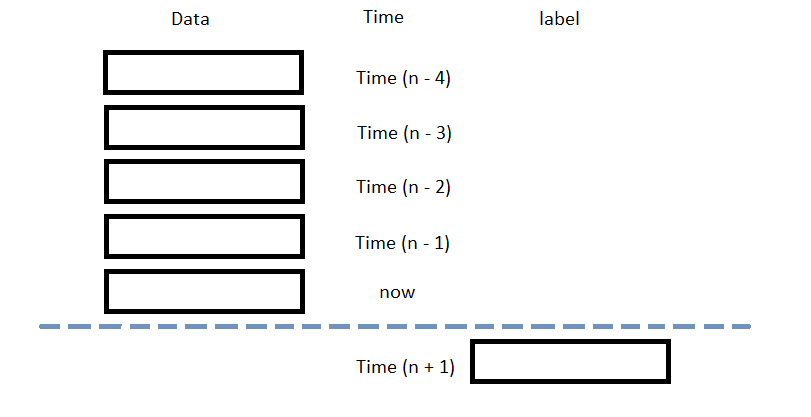# Popular Libraries

## GPlearn

### Introduction

This page explains how to build, train, deploy and store GPlearn models.

### Import Libraries

Import the gplearn and joblib libraries.

from AlgorithmImports import *
from gplearn.genetic import SymbolicRegressor, SymbolicTransformer
import joblib

You need the joblib library to store models.

### Create Subscriptions

In the Initialize method, subscribe to some data so you can train the GPLearn model and make predictions.

self.symbol = self.AddEquity("SPY", Resolution.Daily).Symbol

### Build Models

In this example, build a genetic programming feature transformation model and a genetic programming regression prediction model using the following features and labels:

Data CategoryDescription
FeaturesDaily percent change of the close price of the SPY over the last 5 days
LabelsDaily percent return of the SPY over the next day

The following image shows the time difference between the features and labels:Follow these steps to create a method to build the model:

1. Declare a set of functions to use for feature engineering.
2. function_set = ['add', 'sub', 'mul', 'div',
'sqrt', 'log', 'abs', 'neg', 'inv',
'max', 'min']
3. Call the SymbolicTransformer constructor with the preceding set of functions and then save it as a class variable.
4. self.gp_transformer = SymbolicTransformer(function_set=function_set)
5. Call the SymbolicRegressor constructor to instantiate the regression model.
6. self.model = SymbolicRegressor()

### Train Models

You can train the model at the beginning of your algorithm and you can periodically re-train it as the algorithm executes.

#### Warm Up Training Data

You need historical data to initially train the model at the start of your algorithm. To get the initial training data, in the Initialize method, make a history request.

training_length = 252*2
self.training_data = RollingWindow[float](training_length)
self.training_data.Add(trade_bar.Close)

#### Define a Training Method

To train the model, define a method that fits the model with the training data.

def get_features_and_labels(self, n_steps=5):
training_df = list(self.training_data)[::-1]
daily_pct_change = ((np.roll(training_df, -1) - training_df) / training_df)[:-1]

features = []
labels = []
for i in range(len(daily_pct_change)-n_steps):
features.append(daily_pct_change[i:i+n_steps])
labels.append(daily_pct_change[i+n_steps])
features = np.array(features)
labels = np.array(labels)

return features, labels

def my_training_method(self):
features, labels = self.get_features_and_labels()

# Feature engineering
self.gp_transformer.fit(features, labels)
gp_features = self.gp_transformer.transform(features)
new_features = np.hstack((features, gp_features))

# Fit the regression model with transformed and raw features.
self.model.fit(new_features, labels)

#### Set Training Schedule

To train the model at the beginning of your algorithm, in the Initialize method, call the Train method.

self.Train(self.my_training_method)

To periodically re-train the model as your algorithm executes, in the Initialize method, call the Train method as a Scheduled Event.

# Train the model every Sunday at 8:00 AM
self.Train(self.DateRules.Every(DayOfWeek.Sunday), self.TimeRules.At(8, 0), self.my_training_method)

#### Update Training Data

To update the training data as the algorithm executes, in the OnData method, add the current close price to the RollingWindow that holds the training data.

def OnData(self, slice: Slice) -> None:
if self.symbol in slice.Bars:
self.training_data.Add(slice.Bars[self.symbol].Close)

### Predict Labels

To predict the labels of new data, in the OnData method, get the most recent set of features and then call the predict method.

features, _ = self.get_features_and_labels()

# Get transformed features
gp_features = self.gp_transformer.transform(features)
new_features = np.hstack((features, gp_features))

# Get next prediction
prediction = self.model.predict(new_features)
prediction = float(prediction.flatten()[-1])

You can use the label prediction to place orders.

if prediction > 0:
self.SetHoldings(self.symbol, 1)
elif prediction < 0:
self.SetHoldings(self.symbol, -1)

### Save Models

Follow these steps to save GPLearn models into the Object Store:

1. Set the key names you want to store the models under in the Object Store.
2. transformer_model_key = "transformer"
regressor_model_key = "regressor"
3. Call the GetFilePath method with the keys.
4. transformer_file_name = self.ObjectStore.GetFilePath(transformer_model_key)
regressor_file_name = self.ObjectStore.GetFilePath(regressor_model_key)

This method returns the file paths where the models will be stored.

5. Call the dump method the file paths.
6. joblib.dump(self.gp_transformer, transformer_file_name)
joblib.dump(self.model, regressor_file_name)

If you dump the models using the joblib module before you save the models, you don't need to retrain the models.

You can load and trade with pre-trained GPLearn models that you saved in the Object Store. To load a GPLearn model from the Object Store, in the Initialize method, get the file path to the saved model and then call the load method.

def Initialize(self) -> None:
if self.ObjectStore.ContainsKey(transformer_model_key) and self.ObjectStore.ContainsKey(regressor_model_key):
transformer_file_name = self.ObjectStore.GetFilePath(transformer_model_key)
regressor_file_name = self.ObjectStore.GetFilePath(regressor_model_key)
self.model = joblib.load(regressor_file_name)
The ContainsKey method returns a boolean that represents if transformer_model_key and regressor_model_key are in the Object Store. If the Object Store does not contain the keys, save the model using them before you proceed.